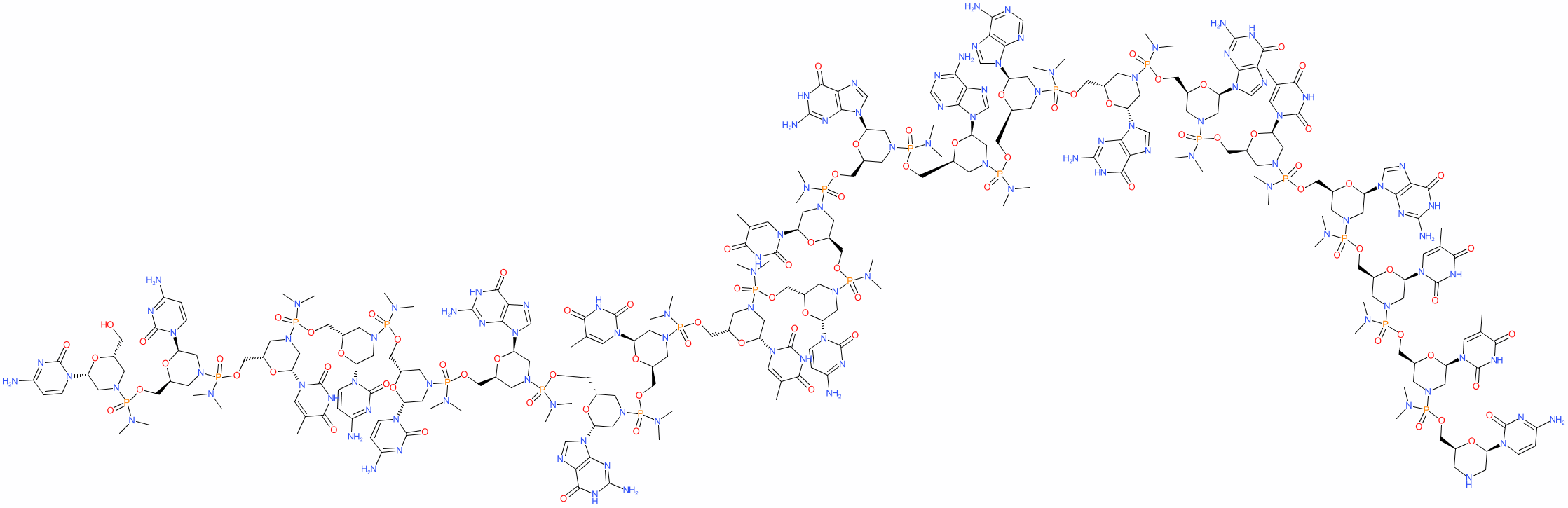#### GtoPdb Ligand ID: 11430

Synonyms: NCNP-01 | NS-065 | Viltepso®
viltolarsen is an approved drug (FDA (2020))
Compound class: Synthetic organic
Comment: Viltolarsen is an antisense phosphorodiamidate morpholino oligomer (PMO) class nucleotide drug. Its abbreviated chemical/nucleotide structure is all-P-ambo-[2',3'-azanediyl-P,2',3'-trideoxy-P-(dimethylamino)-2',3'-seco](2'-N→5')(CCTCCGGTTCTGAAGGTGTTC). Viltolarsen restores the reading frame of certain dystrophin gene mutations and promotes synthesis of a shorter but functional dystrophin protein.
2D StructurePhysico-chemical PropertiesHydrogen bond acceptors 141 Hydrogen bond donors 29 Rotatable bonds 122 Topological polar surface area 2504.15 Molecular weight 6921.36 XLogP -21.82 No. Lipinski's rules broken 3
SMILES / InChI / InChIKeyCanonical SMILES OC[C@H]1O[C@H](CN(C1)P(=O)(N(C)C)OC[C@H]1O[C@H](CN(C1)P(=O)(N(C)C)OC[C@H]1O[C@H](CN(C1)P(=O)(N(C)C)OC[C@H]1O[C@H](CN(C1)P(=O)(N(C)C)OC[C@H]1O[C@H](CN(C1)P(=O)(N(C)C)OC[C@H]1O[C@H](CN(C1)P(=O)(N(C)C)OC[C@H]1O[C@H](CN(C1)P(=O)(N(C)C)OC[C@H]1O[C@H](CN(C1)P(=O)(N(C)C)OC[C@H]1O[C@H](CN(C1)P(=O)(N(C)C)OC[C@@H]1CN(C[C@@H](O1)n1ccc(nc1=O)N)P(=O)(N(C)C)OC[C@H]1O[C@H](CN(C1)P(=O)(N(C)C)OC[C@H]1O[C@H](CN(C1)P(=O)(N(C)C)OC[C@H]1O[C@H](CN(C1)P(=O)(N(C)C)OC[C@H]1O[C@H](CN(C1)P(=O)(N(C)C)OC[C@H]1O[C@H](CN(C1)P(=O)(N(C)C)OC[C@H]1O[C@H](CN(C1)P(=O)(N(C)C)OC[C@H]1O[C@H](CN(C1)P(=O)(N(C)C)OC[C@H]1O[C@H](CN(C1)P(=O)(N(C)C)OC[C@H]1O[C@H](CN(C1)P(=O)(N(C)C)OC[C@H]1O[C@H](CN(C1)P(=O)(N(C)C)OC[C@@H]1CNC[C@@H](O1)n1ccc(nc1=O)N)n1cc(C)c(=O)[nH]c1=O)n1cc(C)c(=O)[nH]c1=O)n1cnc2c1nc(N)[nH]c2=O)n1cc(C)c(=O)[nH]c1=O)n1cnc2c1nc(N)[nH]c2=O)n1cnc2c1nc(N)[nH]c2=O)n1cnc2c1ncnc2N)n1cnc2c1ncnc2N)n1cnc2c1nc(N)[nH]c2=O)n1cc(C)c(=O)[nH]c1=O)n1cc(C)c(=O)[nH]c1=O)n1cc(C)c(=O)[nH]c1=O)n1cnc2c1nc(N)[nH]c2=O)n1cnc2c1nc(N)[nH]c2=O)n1ccc(nc1=O)N)n1ccc(nc1=O)N)n1cc(C)c(=O)[nH]c1=O)n1ccc(nc1=O)N)n1ccc(nc1=O)N Isomeric SMILES OC[C@H]1O[C@H](CN(C1)P(=O)(N(C)C)OC[C@H]1O[C@H](CN(C1)P(=O)(N(C)C)OC[C@H]1O[C@H](CN(C1)P(=O)(N(C)C)OC[C@H]1O[C@H](CN(C1)P(=O)(N(C)C)OC[C@H]1O[C@H](CN(C1)P(=O)(N(C)C)OC[C@H]1O[C@H](CN(C1)P(=O)(N(C)C)OC[C@H]1O[C@H](CN(C1)P(=O)(N(C)C)OC[C@H]1O[C@H](CN(C1)P(=O)(N(C)C)OC[C@H]1O[C@H](CN(C1)P(=O)(N(C)C)OC[C@@H]1CN(C[C@@H](O1)n1ccc(nc1=O)N)P(=O)(N(C)C)OC[C@H]1O[C@H](CN(C1)P(=O)(N(C)C)OC[C@H]1O[C@H](CN(C1)P(=O)(N(C)C)OC[C@H]1O[C@H](CN(C1)P(=O)(N(C)C)OC[C@H]1O[C@H](CN(C1)P(=O)(N(C)C)OC[C@H]1O[C@H](CN(C1)P(=O)(N(C)C)OC[C@H]1O[C@H](CN(C1)P(=O)(N(C)C)OC[C@H]1O[C@H](CN(C1)P(=O)(N(C)C)OC[C@H]1O[C@H](CN(C1)P(=O)(N(C)C)OC[C@H]1O[C@H](CN(C1)P(=O)(N(C)C)OC[C@H]1O[C@H](CN(C1)P(=O)(N(C)C)OC[C@@H]1CNC[C@@H](O1)n1ccc(nc1=O)N)n1cc(C)c(=O)[nH]c1=O)n1cc(C)c(=O)[nH]c1=O)n1cnc2c1nc(N)[nH]c2=O)n1cc(C)c(=O)[nH]c1=O)n1cnc2c1nc(N)[nH]c2=O)n1cnc2c1nc(N)[nH]c2=O)n1cnc2c1ncnc2N)n1cnc2c1ncnc2N)n1cnc2c1nc(N)[nH]c2=O)n1cc(C)c(=O)[nH]c1=O)n1cc(C)c(=O)[nH]c1=O)n1cc(C)c(=O)[nH]c1=O)n1cnc2c1nc(N)[nH]c2=O)n1cnc2c1nc(N)[nH]c2=O)n1ccc(nc1=O)N)n1ccc(nc1=O)N)n1cc(C)c(=O)[nH]c1=O)n1ccc(nc1=O)N)n1ccc(nc1=O)N InChI Key ZVXLKCOOOKREJD-OERAVCCFSA-N
 Download 2D StructureCanonical SMILES Download Isomeric SMILES Download

Molecular structure representations generated using Open Babel